# 机器学习识别验证码

## 就是直接可以用机器学习识别验证码的

2019-03-12 10:52:26 weixin_44274975 阅读数 0
• ###### 机器学习&深度学习系统实战！

数学原理推导与案例实战紧密结合，由机器学习经典算法过度到深度学习的世界，结合深度学习两大主流框架Caffe与Tensorflow，选择经典项目实战人脸检测与验证码识别。原理推导，形象解读，案例实战缺一不可！具体课程...

91课时 988分钟 41001人学习 唐宇迪
免费试看

import pytesseract
from PIL import Image
from selenium import webdriver

browser = webdriver.Chrome(executable_path=’/cdrom/chromedriver’)
browser.get(‘http://piaofang.maoyan.com/?ver=normal’)

money_ele = browser.find_elements_by_css_selector(‘b > i.cs’)
print(money_ele.screenshot(‘ticket.png’))
print(pytesseract.image_to_string(Image.open(‘ticket.png’)))

2017-12-15 11:29:06 shebao3333 阅读数 16348
• ###### 机器学习&深度学习系统实战！

数学原理推导与案例实战紧密结合，由机器学习经典算法过度到深度学习的世界，结合深度学习两大主流框架Caffe与Tensorflow，选择经典项目实战人脸检测与验证码识别。原理推导，形象解读，案例实战缺一不可！具体课程...

91课时 988分钟 41001人学习 唐宇迪
免费试看`Adrian`访问不了用来生成验证码图像的应用程序源代码。因此为了实现上述系统，他只能下载数百个样本图像，人工识别答案，然后作为训练数据来训练他的机器学习系统。## 挑战``````public function __construct() {
/* Characters available in images */
\$this->chars = 'ABCDEFGHJKLMNPQRSTUVWXYZ23456789';

/* Length of a word in an image */
\$this->char_length = 4;

/* Array of fonts. Randomly picked up per character */
\$this->fonts = array(
dirname( __FILE__ ) . '/gentium/GenBkBasR.ttf',
dirname( __FILE__ ) . '/gentium/GenBkBasI.ttf',
dirname( __FILE__ ) . '/gentium/GenBkBasBI.ttf',
dirname( __FILE__ ) . '/gentium/GenBkBasB.ttf',
);``````

## 工具集

• Python 3`Python`是一款有趣的编程语言，有大量用于机器学习和计算机视觉的优秀的库。
• OpenCV`OpenCV`是一个流行的计算机视觉和图像处理框架。 我们将使用`OpenCV`来处理验证码图像。 它有一个`Python``API`，所以我们可以直接从在`Python`中使用它。
• Keras`Keras`是一个用`Python`开发的深度学习框架。 `Keras`的存在简化了深度神经网络的定义、训练和使用，通常你只需要编写少量的代码即可获得一个网络。
• TensorFlow`TensorFlow``Google`的机器学习库。 我们使用`Keras`进行开发，但`Keras`实际上本身并没有实现神经网络的逻辑。 相反，它使用后端的`TensorFlow`库来完成繁重的工作。

## 创建数据集## 简化问题## 建立和培养神经网络`Keras`中定义这个神经网络只需要几行代码：

``````# Build the neural network!
model = Sequential()

# First convolutional layer with max pooling

# Second convolutional layer with max pooling

# Hidden layer with 500 nodes

# Output layer with 32 nodes (one for each possible letter/number we predict)

# Ask Keras to build the TensorFlow model behind the scenes

``````# Train the neural network
model.fit(X_train, Y_train, validation_data=(X_test, Y_test), batch_size=32, epochs=10, verbose=1)``````

## 使用训练好的模型来识别验证码

1. 从使用此`WordPress`插件的网站抓取真实的验证码图像。
2. 使用我们用来创建训练数据集的相同方法，将验证码图像分解为四个独立的字母图像。
3. 让神经网络对每个字母图像做一个单独的预测。
4. 使用四个预测字母作为验证码的答案。
5. 狂欢吧！## python 基于机器学习识别验证码

2018-04-28 00:06:59 Neleuska 阅读数 19647
• ###### 机器学习&深度学习系统实战！

数学原理推导与案例实战紧密结合，由机器学习经典算法过度到深度学习的世界，结合深度学习两大主流框架Caffe与Tensorflow，选择经典项目实战人脸检测与验证码识别。原理推导，形象解读，案例实战缺一不可！具体课程...

91课时 988分钟 41001人学习 唐宇迪
免费试看

## 1、背景

验证码自动识别在模拟登陆上使用的较为广泛，一直有耳闻好多人在使用机器学习来识别验证码，最近因为刚好接触这方面的知识，所以特定研究了一番。发现网上已有很多基于machine learning的验证码识别，本文主要参考几位大牛的研究成果，集合自己的需求，进行改进、学习。

## 2、基本工具

python 3.5 + pycharm

Pillow、sklearn、numpy及其他子模块

①验证码清理并生成训练集样本

②验证码特征提取

③拟合识别模型

④识别模型测试

## 4、关于数据集

没有特意网上找python的生成脚本，用了一个java的验证码生成脚本。验证码是数字+大写字母+小写字母的组合，即[0-9]+[A-Z]+[a-z]。文件名是验证码的正确数字标签，实例如下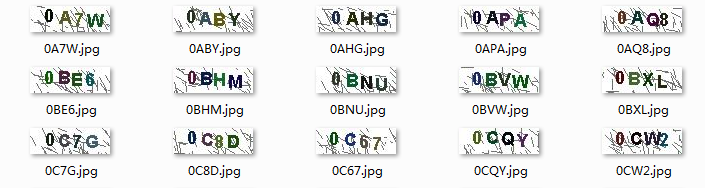①训练集（training set）:10000张验证码

②测试集（test set）：100张验证码

③验证集（validation set）：100张验证码

## 5、验证码清理并生成训练集样本

（1）读取图片

首先读取该文件路径下的所有图片文件名称，并逐张打开。返回结果image_array，每一个元素类型为“<class 'PIL.JpegImagePlugin.JpegImageFile'>”。

``````def read_captcha(path):
image_array = []
image_label = []
for file in file_list:
image = Image.open(path + '/' + file)   # 打开图片
file_name = file.split(".")  #获取文件名，此为图片标签
image_array.append(image)
image_label.append(file_name)
return image_array, image_label``````

（2）图像粗清理

图像粗清理包括以下步骤：

step 1：原始图像是RGB图像，即维度为 (26, 80, 3)。将其转换为灰度图像，维度变为(26, 80)。灰度图像：step 2：对于将要识别的验证码，显然，里面出现了很多用于干扰作用的灰色线条。博主通过设定灰度阈值（默认100），对图像中大于阈值的像素，赋值为255（灰度图像中像素值范围是0~255，其中255是白色，0是黑色）。发现对于此类型的验证码，这种方法很实用有木有。``````def image_transfer(image_arry):
"""
:param image_arry:图像list，每个元素为一副图像
:return: image_clean:清理过后的图像list
"""
image_clean = []
for i, image in enumerate(image_arry):
image = image.convert('L') # 转换为灰度图像，即RGB通道从3变为1
im2 = Image.new("L", image.size, 255)
for y in range(image.size): # 遍历所有像素，将灰度超过阈值的像素转变为255（白）
for x in range(image.size):
pix = image.getpixel((x, y))
if int(pix) > threshold_grey:  # 灰度阈值
im2.putpixel((x, y), 255)
else:
im2.putpixel((x, y), pix)
image_clean.append(im2)
return image_clean``````

（3）图像细清理

仅仅通过粗清理的办法，无法完全去除所有噪声点。此处引入了更细粒度的清理方法，参考这位大牛的清理方法。

step 1：找出图像中所有的孤立点；

step 2：计算黑色点近邻9宫格中黑色点个数，若小于等于2个，那么认为该点为噪声点；

step 3：去除所有噪声点。

经过细清理后，虽然可以看到还存在一个噪声点，但效果其实很不错了。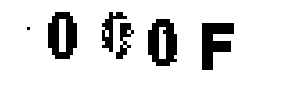（4）单字符图像切割

去除孤立点后，我们还是没法一下子就识别出这四个字符，需要对经过处理后的图片进行切分。（其实可以使用deep learning的方法进行识别，但本文仅介绍基于machine learning的识别方法）

切割方式主要有一下步骤：

step 1：找出图片中所有分离图像的开始结束位置。遍历width&height，当每出现一个黑色点，记为该字符开始位置；当新的一列出现全白色点，那么记为结束位置。

``[(8, 9), (14, 22), (29, 38), (42, 50), (57, 66)]``

step 2：尽管经过清理后，还是可能存在噪声点。在找到所有切割开始结束位置后，计算并选出（结束值-开始值）最大的切割位置。

``[(14, 22), (29, 38), (42, 50), (57, 66)]``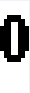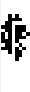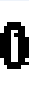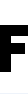code：

``````def image_split(image):
"""
:param image:单幅图像
:return:单幅图像被切割后的图像list
"""
inletter = False    #找出每个字母开始位置
foundletter = False #找出每个字母结束位置
start = 0
end = 0
letters = []    #存储坐标
for x in range(image.size):
for y in range(image.size):
pix = image.getpixel((x, y))
if pix != True:
inletter = True
if foundletter == False and inletter == True:
foundletter = True
start = x
if foundletter == True and inletter == False:
foundletter = False
end = x
letters.append((start, end))
inletter = False

# 因为切割出来的图像有可能是噪声点
# 筛选可能切割出来的噪声点,只保留开始结束位置差值最大的位置信息
subtract_array = []    # 存储 结束-开始 值
for each in letters:
subtract_array.append(each-each)
reSet = sorted(subtract_array, key=lambda x:x, reverse=True)[0:image_character_num]
letter_chioce = []    # 存储 最终选择的点坐标
for each in letters:
if int(each - each) in reSet:
letter_chioce.append(each)

image_split_array = []    #存储切割后的图像
for letter in letter_chioce:
im_split = image.crop((letter, 0, letter, image.size)) # (切割的起始横坐标，起始纵坐标，切割的宽度，切割的高度)
im_split = im_split.resize((image_width, image_height)) # 转换格式
image_split_array.append(im_split)

return image_split_array[0:int(image_character_num)]``````

（5）保存到训练集

将按上述方法切分后的单个数字、字母，保存到新建的文件夹里，专门用来作为模型的训练集。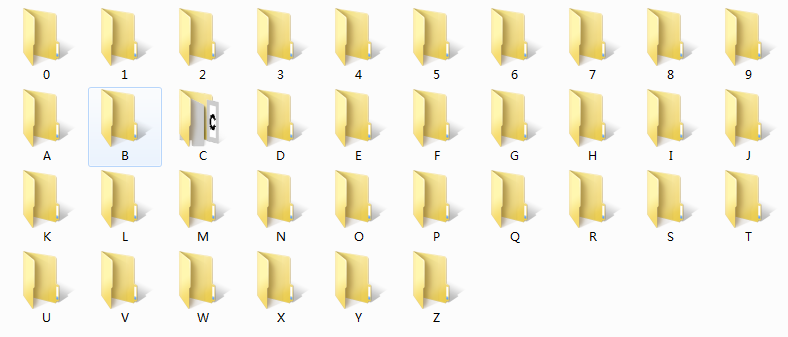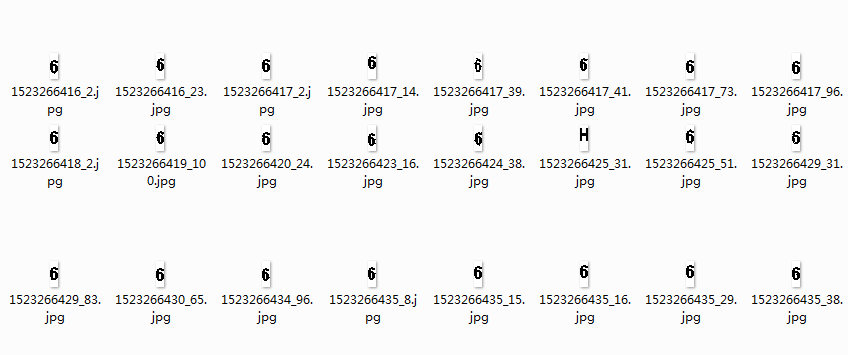## 6、特征提取

特征提取是针对每一个切割出后的单个字符，如6。此处构建特征的方法较为简单，统计每个字符图像每一行像素值为黑色的总和（灰度值为0），加上每一列像素值为黑色的总和。因为我们切割后的图像大小为8*26（width*height），故特征个数为34=8+26。当然此处其实可以把单字符图像按像素值展开为一个208=8*26的向量，以此作为特征向量，也是可以的。示例结果如下所示：

``feature vector: [7, 11, 13, 4, 4, 13, 11, 7, 0, 0, 0, 0, 0, 4, 6, 4, 6, 6, 6, 6, 6, 6, 6, 4, 6, 4, 0, 0, 0, 0, 0, 0, 0, 0]``

聪明的你可能会发现了一个致命的问题，如果使用新类型/不同像素大小的验证码来做处理和特征提取，那程序不就报错了？我们已经在切割的步骤后面加上像素大小的转换：

``im_split = im_split.resize((image_width, image_height)) # 转换格式，im_split为切割后的图像，image_width为目标像素宽度，iamge_height为目标像素高度``

当然，在读取图像的时候就转换格式也是可以的~

code：

``````def feature_transfer(image):
"""
:param image （图像list）
:return:feature （特征list）
"""
image = image.resize((image_width, image_height)) #标准化图像格式

feature = []#计算特征
for x in range(image_width):#计算行特征
feature_width = 0
for y in range(image_height):
if image.getpixel((x, y)) == 0:
feature_width += 1
feature.append(feature_width)

for y in range(image_height): #计算列特征
feature_height = 0
for x in range(image_width):
if image.getpixel((x, y)) == 0:
feature_height += 1
feature.append(feature_height)
# print('feature length :',len(feature))
print("feature vector:",feature)
return feature``````

## 7、训练识别模型

关于训练识别模型，使用全量学习的方式，训练集用于拟合模型，测试集用于测试模型效果。本博客对比了SVC、random forest。

关于SVC的参数，使用了不同kernel（线性核、高斯核），以及在一定范围内修改了正则项C，但测试效果不十分理想。对于正则项C，位于SVM模型的目标函数位置，当C越大时，模型对误分类的惩罚增大，反之，减少。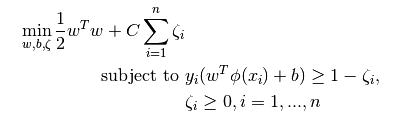关于随机森林的参数，调整了树的深度、每个节点分支需要的最少样本数，尽量简化了每棵树的结构。效果较SVC好。

得到的结果如下

 model parameters training accuracy test accuracy SVC(linear) C=1.0 0.9125 0.65 SVC(rbf) C=1.0 0.9055 0.55 Random Foest max_depth=10, min_sample_split=10 0.9420 0.75

code：

``````def trainModel(data, label):
print("fit model >>>>>>>>>>>>>>>>>>>>>>")

# svc_rbf = svm.SVC(decision_function_shape='ovo',kernel='rbf')    # rbf核svc
# svc_linear = svm.SVC(decision_function_shape='ovo',kernel='linear')    #linear核svc
rf = RandomForestClassifier(n_estimators=100, max_depth=10,min_samples_split=10, random_state=0)    #随机森林
scores = cross_val_score(rf, data, label,cv=10)    #交叉检验，计算模型平均准确率
print("rf: ",scores.mean())
rf.fit(data, label)    # 拟合模型

joblib.dump(rf, model_path) # 模型持久化，保存到本地
print("model save success!")

return rf``````

当训练样本非常大，如上千万/亿的时候，若使用传统machine learning的全量学习方法，需要消耗大量内容，对个人用户并不友好，此时可以引入增量学习。类似于训练neural network，每次训练只使用一个batch的小数据集，经过多次迭代，可达到非常robust的效果。

scikit-learn中支持SGD、Naive Bayes等分类模型的增量学习。通过迭代的方式，每次生成小数据集batch，使用partial_fit()方法训练模型。

scikit-learn 0.19.1 支持如下模型的增量学习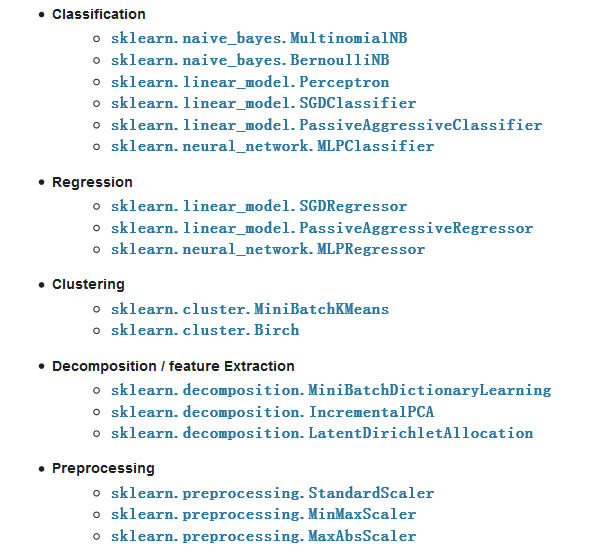## 8、模型测试效果

得到的结果如下

 model parameters training accuracy test accuracy SVC(linear) C=1.0 0.9125 0.65 SVC(rbf) C=1.0 0.9055 0.55 Random Foest max_depth=10, min_sample_split=10 0.9420 0.75

## 9、识别预测流程

经过上述步骤，我们已经训练好了一个具有一定识别验证码能力的模型，为了能让模型自动化实现输入验证码文件，输出验证码识别结果，流程如下：

①读取将要识别的验证码文件

②验证码粗清理。将灰度值小于阈值的像素值转化为255。

③验证码细清理。找出所有孤立的噪声点，并将该像素值转化为255。

④字符切割。找出所有字符的开始结束位置，并切割出4幅图像。

⑤图像特征提取。对于4幅图像中的每一幅，分别从行、列统计其灰度值为0（黑色）的和，构建4个特征向量。

⑥识别。读取训练好的模型，分别对4个特征向量进行识别，得到4个预测结果。

⑦输出。将识别出的4个字符结果，串起来，并输出到结果文件。

code：

``````#-*- coding:utf-8 -*

import os
from sklearn.externals import joblib

#验证码数据清洗
def clean():
#验证码清理
print("待测试的验证码数量：", len(image_array))
image_clean = image_process.image_transfer(image_array) #转换成灰度图像，并去除背景噪声
image_array = [] #[[im_1_1,im_1_2,im_1_3,im_1_4],[im_2_1,im_2_2,im_2_3,im_2_4],...]
for each_image in image_clean:
image_out = image_process.get_clear_bin_image(each_image) #转换为二值图片，并去除剩余噪声点
split_result = image_process.image_split(image_out) #切割图片
image_array.append(split_result)
return image_array, image_label

#特征矩阵生成
def featrue_generate(image_array):
feature = []
for num, image in enumerate(image_array):
feature_each_image = []
for im_meta in image:
fea_vector = image_feature.feature_transfer(im_meta)
# print('label: ',image_label[num])
# print(feature)
feature_each_image.append(fea_vector)
# print(fea_vector)
# print(len(feature_each_image))
if len(feature_each_image) == 0:
feature_each_image = [*(image_width+image_height)]*int(image_character_num)
# print(feature_each_image)
feature.append(feature_each_image)
print("预测数据的长度:", len(feature))
print("预测数据特征示例:", feature)
return feature

#将结果写到文件
def write_to_file(predict_list):
file_list = os.listdir(test_data_path)
with open(output_path, 'w') as f:
for num, line in enumerate(predict_list):
if num == 0:
f.write("file_name\tresult\n")
f.write(file_list[num] + '\t' + line + '\n')
print("结果输出到文件：", output_path)

def main():
#验证码清理
image_array, image_label = clean()
#特征处理
feature = featrue_generate(image_array)
#预测
predict_list = []
acc = 0
# print("预测错误的例子：")
for num, line in enumerate(feature):
# print(line)
predict_array = model.predict(line)
predict = ''.join(predict_array)
predict_list.append(predict)
if predict == image_label[num]:
acc += 1
else:
pass
print("-----------------------")
print("actual:",image_label[num])
print("predict:", predict)
print("测试集预测acc：", acc/len(image_label))
#输出到文件
write_to_file(predict_list)

if __name__ == '__main__':
main()``````

## 10、总结

关于上述机器学习的验证码识别，只是作了一个简单例子的过程演示。仅仅是针对某种特定类型的验证码，若换成其他类型的验证码做测试，不能保证识别的准确率。

这就是传统机器学习的不足：需要人工做数据清理和提炼特征。有个办法可以解决这种繁琐的数据清理，以及人工提取验证码特征的缺点，那就是深度学习的方法。

博主使用深度学习的循环神经网络，训练了一个识别模型，具体请跳转到这里

## 11、相关博客&文献

https://www.cnblogs.com/TTyb/p/6156395.html?from=timeline&isappinstalled=0

https://www.cnblogs.com/beer/p/5672678.html

## 机器学习之验证码识别

2018-06-29 09:59:13 Python1991 阅读数 1161
• ###### 机器学习&深度学习系统实战！

数学原理推导与案例实战紧密结合，由机器学习经典算法过度到深度学习的世界，结合深度学习两大主流框架Caffe与Tensorflow，选择经典项目实战人脸检测与验证码识别。原理推导，形象解读，案例实战缺一不可！具体课程...

91课时 988分钟 41001人学习 唐宇迪
免费试看

1. ＃ - * - 编码：utf-8 - * -
2. 导入请求
3. 进口时间
4. ＃文件下载，主要下载训练集
6. url = 'http: //smart.gzeis.edu.cn:8081/Content/ AuthCode.aspx'
7. res = requests.get（url，stream = True
8. 开放（u'J：/数据分析学习/蟒/机器学习之验证码识别/pics/%s.jpg”％（pic_name），把wb F：
9. 对于组块 res.iter_content（CHUNK_SIZE = 1024）：
10. 如果大块：
11. f.write（块）
12. f.flush（）
13. f.close（）
14. if __name__ == '__main__':
15. for i in xrange(100):
16. pic_name = int(time.time()*1000000)1. # -*- coding: utf-8 -*-
2. from PIL import Image,ImageEnhance
3. from PIL import *
4. import time
5. # 图片切割
6. def segment(im):
7. s = 12
8. w = 40
9. h = 81
10. t = 0
11. im_new = []
12. for i in range(4):
13. im1 = im.crop((s+w*i,t,s+w*(i+1),h))
14. im_new.append(im1)
15. return im_new
16. # 图片预处理，二值化，图片增强
17. def imgTransfer(f_name):
18. im = Image.open(f_name)
19. im = im.filter(ImageFilter.MedianFilter())
20. #enhancer = ImageEnhance.Contrast(im)
21. #im = enhancer.enhancer(1)
22. im = im.convert('L')
23. return im
24. def cutPictures(img):
25. im = imgTransfer(img)
26. pics = segment(im)
27. for pic in pics:
28. pic.save(u'J:/数据分析学习/python/机器学习之验证码识别/test/%s.jpeg'%(int(time.time()*1000000)),'jpeg')
29. # 读取某文件夹下的所有图片
30. import os
31. def getAllImages(folder):
32. assert os.path.exists(folder)
33. assert os.path.isdir(folder)
34. imageList = os.listdir(folder)
35. imageList = [os.path.abspath(item) for item in imageList if os.path.isfile(os.path.join(folder, item))]
36. return imageList
37. if __name__ == '__main__':
38. files_name = getAllImages(u'J:/数据分析学习/python/机器学习之验证码识别/pics//')
39. for i in files_name:
40. #cutPictures()
41. files = i.replace('\\','/')
42. s = files.split('/')
43. name = ''
44. for j in s[:-1]:
45. name = name + j + '/'
46. name = name + 'pics/' + s[-1]
47. cutPictures(name)1. # -*- coding: utf-8 -*-
2. """
3. Created on Thu Mar 23 14:19:13 2017
4. 对切割后的图片进行分类，及0-9
5. @author: onlyyo
6. """
7. import sys
8. sys.path.append('C:\Users\onlyyo\Desktop\pytesseract-0.1.6\src')
9. sys.path.append('C:\Python27\Lib\site-packages\pytesser')
10. from pytesser import *
11. from pytesseract import *
12. import pytesseract
13. from PIL import Image
14. import os
15. import shutil
16. #ocr图像识别
17. def ocr(img):
18. try:
19. img = Image.open(img)
20. rs = image_to_string(img)
21. except:
22. return 'none'
23. return rs
24. #使用ocr进行训练的预分类
25. def category(originfile,dirs,filename):
26. if not os.path.exists(dirs):
27. os.makedirs(dirs)
28. shutil.copyfile(originfile,dirs+filename)
29. if __name__ == '__main__':
30. dirs = u'J:/数据分析学习/python/机器学习之验证码识别/test/'
31. # 将ocr识别的文件按照数组编号存放在相应的文件夹中
32. for fr in os.listdir(dirs):
33. f = dirs+fr
34. if f.rfind(u'.DS_Store') == -1:
35. rs = ocr(f)
36. if '|' not in rs and '*' not in rs :
37. if '?' not in rs and '<'not in rs and '>' not in rs:
38. category(f,u'J:/数据分析学习/python/机器学习之验证码识别/category/%s/'%rs,fr)1. # -*- coding: utf-8 -*-
2. """
3. Created on Thu Mar 23 15:46:59 2017
4. @author: onlyyo
5. 批量将切割后并且已经分好类的图像，得到的图片进行二值化处理，变成像素值，然后保存在TXT文件下
6. """
7. from PIL import Image
8. import numpy as np
9. import os
10. # 特征提取，获取图像二值化数学值
11. def getBinaryPix(im):
12. im = Image.open(im)
13. img = np.array(im)
14. rows,cols = img.shape
15. for i in range(rows):
16. for j in range(cols):
17. if (img[i,j]<= 128):
18. img[i,j] = 0
19. else:
20. img[i,j] = 1
21. binpix = np.ravel(img)
22. return binpix
23. def getfiles(dirs):
24. fs = []
25. for fr in os.listdir(dirs):
26. f = dirs + fr
27. if f.rfind(u'.DS_Store') == -1:
28. fs.append(f)
29. return fs
30. def writeFile(content):
31. with open(u'J:/数据分析学习/python/机器学习之验证码识别/traindata/train_data.txt','a+') as f:
32. f.write(content)
33. f.write('\n')
34. f.close()
35. if __name__ == '__main__':
36. dirs = u'J:/数据分析学习/python/机器学习之验证码识别/category/%s/'
37. for i in range(9):
38. for f in getfiles(dirs %(i)):
39. pixs = getBinaryPix(f).tolist()
40. pixs.append(i)
41. pixs = [str(i) for i in pixs]
42. content = ','.join(pixs)
43. writeFile(content)

1. # -*- coding: utf-8 -*-
2. """
3. Created on Thu Mar 23 16:18:39 2017
4. @author: onlyyo
5. 根据之前得到的验证码像素数组，以及他们的标签，用SVM对其进行模型训练
6. """
7. from sklearn.svm import SVC
8. from sklearn import grid_search
9. import numpy as np
10. import numpy as np
11. from sklearn import cross_validation as cs
12. from sklearn.externals import joblib
14. import warnings
15. import time
18. return dataset
19. # 交叉验证
20. def cross_validation():
22. row,col = dataset.shape
23. X = dataset[:,:col-1]
24. Y = dataset[:,-1]
25. clf = SVC(kernel='rbf',C=1000)
26. clf.fit(X,Y)
27. scores = cs.cross_val_score(clf,X,Y,cv=5)
28. print "Accuracy: %0.2f (+- %0.2f)" % (scores.mean(),scores.std())
29. return clf
30. t0 = time.time()
31. cross_validation()
32. #print "fit time:",round(time.time()-t0,3),"s"
33. def searchBestParameter():
34. parameters = {'kernel':('linear','poly','rbf','sigmoid'),'C':[1,100]}
36. row,col = dataset.shape
37. X = dataset[:,:col-1]
38. Y = dataset[:,-1]
39. svr = SVC()
40. clf = grid_search.GridSearchCV(svr,parameters)
41. clf.fit(X,Y)
42. print clf.best_params_
43. #searchBestParameter()
44. print "fit time:",round(time.time()-t0,3),"s"

1. # -*- coding: utf-8 -*-
2. """
3. Created on Thu Mar 23 17:20:09 2017
4. @author: onlyyo
5. 最后一步，对于要测试的验证码处理，然后进行预测，输出结果
6. """
7. from split_pic import *
8. from write_img import *
9. import os
10. from cross_svc import cross_validation
11. def cutPictures2(name):
12. im = imgTransfer(name)
13. pics = segment(im)
14. for pic in pics:
15. pic.save(u'J:/数据分析学习/python/机器学习之验证码识别/test_picture/%s.jpeg'%(int(time.time()*1000000)),'jpeg')
17. #
18. cutPictures2(name) #切割图片
19. dirs = u'J:/数据分析学习/python/机器学习之验证码识别/test_picture/'
20. fs = os.listdir(dirs) # 获取图片名称
21. clf = cross_validation()
22. predictValue = []
23. for fname in fs:
24. fn = dirs + fname
25. binpix = getBinaryPix(fn)
26. predictValue.append(clf.predict(binpix))
27. predictValue = [str(int(i)) for i in predictValue]
28. 打印 “图片编号为：”“”. join（predictValue）
29. name = u'J：/数据分析学习/ python /机器学习之验证码识别/8473.jpg'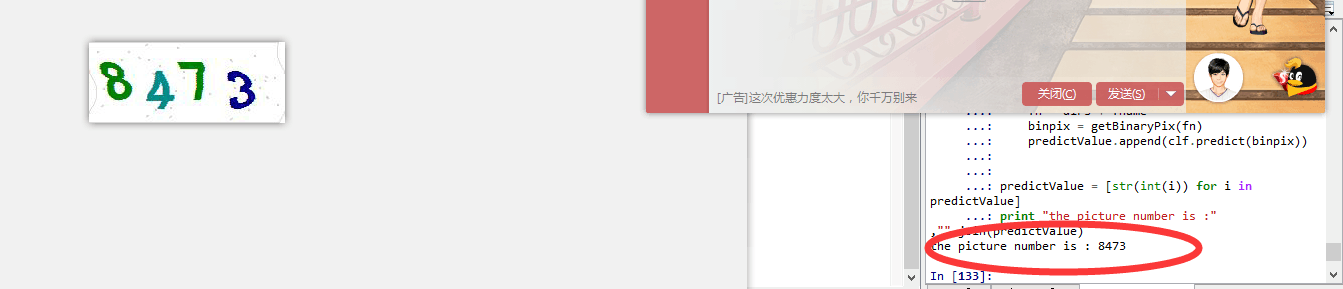## 利用机器学习识别验证码（从0到1）

2019-06-07 22:13:07 weixin_43582101 阅读数 809
• ###### 机器学习&深度学习系统实战！

数学原理推导与案例实战紧密结合，由机器学习经典算法过度到深度学习的世界，结合深度学习两大主流框架Caffe与Tensorflow，选择经典项目实战人脸检测与验证码识别。原理推导，形象解读，案例实战缺一不可！具体课程...

91课时 988分钟 41001人学习 唐宇迪
免费试看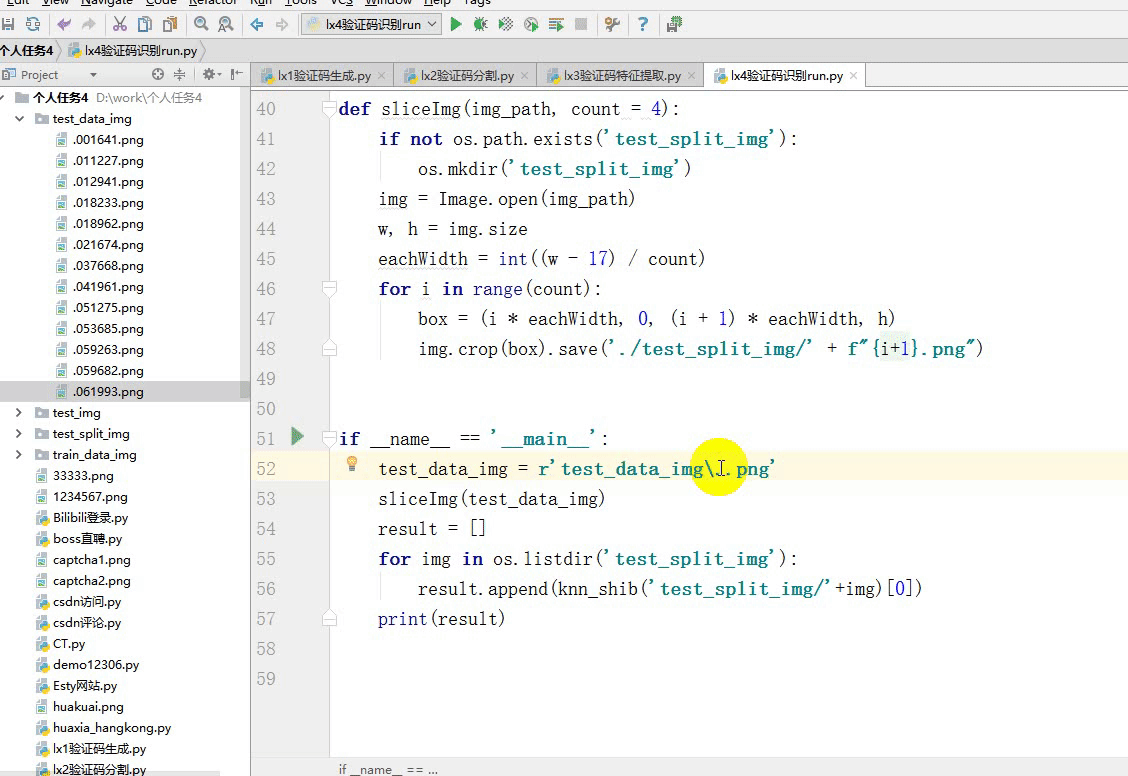## 一：生成验证码：

``````import random,os
from PIL import ImageFont,Image,ImageDraw,ImageFilter
def auth_code():
size = (140,40)                             #图片大小
font_list = list("0123456789")             #验证码范围
c_chars = "  ".join(random.sample(font_list,4))  # 4个+中间加个俩空格
print(c_chars)
img = Image.new("RGB",size,(33,33,34))      #RGB颜色
draw = ImageDraw.Draw(img)                  #draw一个
font = ImageFont.truetype("arial.ttf", 23)      #字体
draw.text((5,4),c_chars,font=font,fill="white") #字颜色
params = [1 - float(random.randint(1, 2)) / 100,
0,
0,
0,
1 - float(random.randint(1, 10)) / 100,
float(random.randint(1, 2)) / 500,
0.001,
float(random.randint(1, 2)) / 500
]
img = img.transform(size, Image.PERSPECTIVE, params)
img = img.filter(ImageFilter.EDGE_ENHANCE_MORE)
img.save(f'./test_img/{c_chars}.png')

if __name__ == '__main__':
if not os.path.exists('./test_img'):
os.mkdir('./test_img')
while True:
auth_code()
if len(os.listdir('./test_img'))>=3000:     # 你改成 10000就行了
#我这个电脑太老了。
break
``````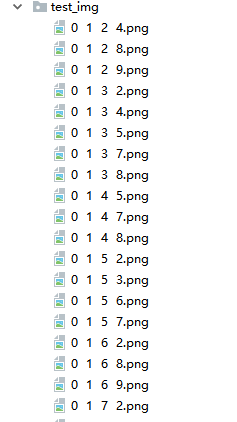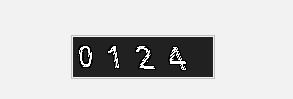## 二：验证码分割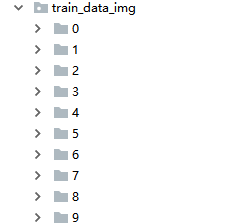``````import os
from PIL import Image
from sklearn.externals import joblib
import time
img_array = []
img_lable = []
file_list = os.listdir('./test_img')
for file in file_list:
try:
image = file
img_array.append(image)
except:
print(f'{file}:图像已损坏')
os.remove('./test_img/'+file)
return img_array

def sliceImg(img_path, count = 4):
if not os.path.exists('train_data_img'):
os.mkdir('train_data_img')
for i in range(10):
if not os.path.exists(f'train_data_img/{i}'):
os.mkdir(f'train_data_img/{i}')
img = Image.open('./test_img/'+img_path)
w, h = img.size
eachWidth = int((w - 17) / count)
img_path = img_path.replace(' ', '').split('.')

for i in range(count):
box = (i * eachWidth, 0, (i + 1) * eachWidth, h)
img.crop(box).save(f'./train_data_img/{img_path[i]}/'+img_path[i]+ str(time.time()) + ".png")

if __name__ == '__main__':
for i in img_array:
print(i)
sliceImg(i)
``````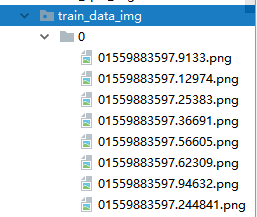## 三：验证码特征提取

``````from PIL import Image
import numpy as np
import os
from sklearn.neighbors import KNeighborsClassifier as knn
def img2vec(fname):
'''将图片转为向量'''
im = Image.open(fname).convert('L')
im = im.resize((30,30))
tmp = np.array(im)
vec = tmp.ravel()
return vec
``````

``````tarin_img_path = 'train_data_img'
def split_data(paths):
X = []
y = []
for i in os.listdir(tarin_img_path):
path = os.path.join(tarin_img_path, i)
fn_list = os.listdir(path)
for name in fn_list:
y.append(name)
X.append(img2vec(os.path.join(path,name)))
return X, y                 # x向量   y标签
``````

``````def knn_clf(X_train,label):
'''构建分类器'''
clf = knn()
clf.fit(X_train,label)
return clf
``````

``````def knn_shib(test_img):
X_train,y_label = split_data(tarin_img_path)
clf = knn_clf(X_train,y_label)
result = clf.predict([img2vec(test_img)])
return result
``````

## 四：验证码识别

``````import random,time
import os
from PIL import ImageFont,Image,ImageDraw,ImageFilter
def auth_code():
size = (140,40)                             #图片大小
font_list = list("0123456789")             #验证码范围
c_chars = "  ".join(random.sample(font_list,4))  # 4个+中间加个俩空格
print(c_chars)
img = Image.new("RGB",size,(33,33,34))      #RGB颜色
draw = ImageDraw.Draw(img)                  #draw一个
font = ImageFont.truetype("arial.ttf", 23)      #字体
draw.text((5,4),c_chars,font=font,fill="white") #字颜色
params = [1 - float(random.randint(1, 2)) / 100,
0,
0,
0,
1 - float(random.randint(1, 10)) / 100,
float(random.randint(1, 2)) / 500,
0.001,
float(random.randint(1, 2)) / 500
]
img = img.transform(size, Image.PERSPECTIVE, params)
img = img.filter(ImageFilter.EDGE_ENHANCE_MORE)
random_name = str(time.time())[-7:]
img.save(f'./test_data_img/{random_name}.png')
if __name__ == '__main__':
if not os.path.exists('./test_data_img'):
os.mkdir('./test_data_img')
while True:
auth_code()
if len(os.listdir('./test_data_img'))>=30:
break
``````

``````from lx3验证码特征提取 import *
from lx2验证码分割     import *

def sliceImg(img_path, count = 4):
if not os.path.exists('test_split_img'):
os.mkdir('test_split_img')
img = Image.open(img_path)
w, h = img.size
eachWidth = int((w - 17) / count)
for i in range(count):
box = (i * eachWidth, 0, (i + 1) * eachWidth, h)
img.crop(box).save('./test_split_img/' + f"{i+1}.png")

if __name__ == '__main__':
test_data_img = r'test_data_img\.059682.png'
sliceImg(test_data_img)
result = []
for img in os.listdir('test_split_img'):
result.append(knn_shib('test_split_img/'+img))
print(result)
``````